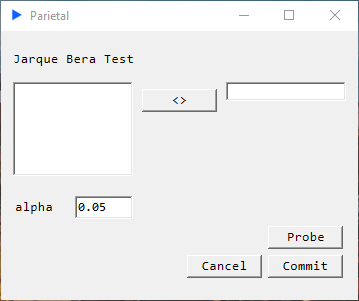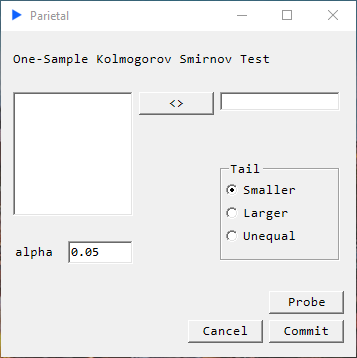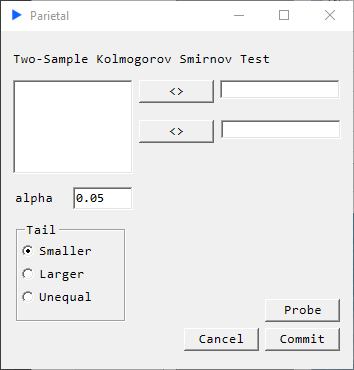# Distribution Tests

## Chi-Square Goodness of Fit Test###### Description#

Determines if the sample derives from a population with a normal distribution the mean and variance of which are calculated from the sample itself.

$H_{0}:$ Data in sample derives from a population with a normal distribution

$H_{1}:$ Data in sample does not derive from a population with a normal distribution

##### Returns#
• p-value
• decision
• Chi-Sq statistic

## Jarque Bera Test###### Description#

Uses the sample skewness and sample kurtosis to determine if the sample derives from a normal distribution of unknown mean and variance.

$H_{0}:$ Data in sample derives from a population with a normal distribution

$H_{1}:$ Data in sample does not derive from a population with a normal distribution

• p-value
• decision
• JB statistic

## One Sample Kolmogorov Smirnov Test (KS1)###### Description#

Determines if the sample derives from a population with a standard normal distribution.

$H_{0}:$ Population CDF of the sample is the same as std normal CDF

TailAlt. Hypothesis
$smaller\hspace{1mm}$Population CDF of the sample is smaller than std normal CDF
$larger\hspace{1mm}$Population CDF of the sample is larger than std normal CDF
$unequal\hspace{1mm}$Population CDF of the sample is not same as std normal CDF
• p-value
• decision
• KS statistic

## Two Sample Kolmogorov Smirnov Test (KS2)###### Description#

Determine if the two samples derive from the same distribution but make no assumptions about what that distribution is.

$H_{0}: \text{population CDF of the sample 1 is the same as population CDF of sample 2}$

TailAlt. Hypothesis
$smaller\hspace{1mm}$Population CDF of the sample 1 is smaller than population CDF of sample 2
$larger\hspace{1mm}$Population CDF of the sample 1 is larger than population CDF of sample 2
$unequal\hspace{1mm}$Population CDF of the sample 1 is not same as population CDF of sample 2
• p-value
• decision
• KS statistic